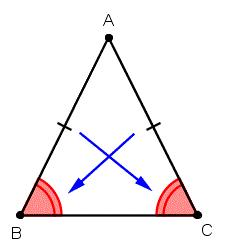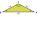# Ratio iso triangle

The ratio of the sides of an isosceles triangle is 7:6:7 Find the base angle to the nearest answer correct to 3 significant figure.

A =  64.6231 °

### Step-by-step explanation:

Try calculation via our triangle calculator.Did you find an error or inaccuracy? Feel free to write us. Thank you!Tips to related online calculators
Check out our ratio calculator.

#### You need to know the following knowledge to solve this word math problem:

We encourage you to watch this tutorial video on this math problem:

## Related math problems and questions:

• Isosceles triangle 8If the rate of the sides an isosceles triangle is 7:6:7, find the base angle correct to the nearest degree.
• Isosceles triangle 10In an isosceles triangle, the equal sides are 2/3 of the length of the base. Determine the measure of the base angles.
• Roof angleThe roof of the house has the shape of an isosceles triangle with arms 4 m long and the size of the base 6 m. How big an angle alpha does its roof make?
• The aspect ratioThe aspect ratio of the rectangular triangle is 13: 12: 5. Calculate the internal angles of the triangle.
• Right triangle eq2Find the lengths of the sides and the angles in the right triangle. Given area S = 210 and perimeter o = 70.
• Trapezoid MOThe rectangular trapezoid ABCD with the right angle at point B, |AC| = 12, |CD| = 8, diagonals are perpendicular to each other. Calculate the perimeter and area of ​​the trapezoid.
• Isosceles triangleWhat are the angles of an isosceles triangle ABC if its base is long a=5 m and has an arm b=4 m.
• Sphere in coneA sphere is inscribed in the cone (the intersection of their boundaries consists of a circle and one point). The ratio of the surface of the ball and the contents of the base is 4: 3. A plane passing through the axis of a cone cuts the cone in an isoscele
• Largest angle of the triangleCalculate the largest angle of the triangle whose sides have the sizes: 2a, 3/2a, 3a
• Ratio in trapeziumThe height v and the base a, c in the trapezoid ABCD are in the ratio 1: 6: 3, its content S = 324 square cm. Peak angle B = 35 degrees. Determine the perimeter of the trapezoid
• Triangle in a squareIn a square ABCD with side a = 6 cm, point E is the center of side AB, and point F is the center of side BC. Calculate the size of all angles of the triangle DEF and the lengths of its sides.
• TreeBetween points A and B is 50m. From A we see a tree at an angle 18°. From point B we see the tree in three times bigger angle. How tall is a tree?
• Angle in RTDetermine the size of the smallest internal angle of a right triangle whose sides constitutes sizes consecutive members of arithmetic progressions.
• ISO triangleCalculate the area of an isosceles triangle KLM if its sides' length is in the ratio k:l:m = 4:4:3 and has perimeter 377 mm.
• Traffic signThere is a traffic sign for climbing on the road with an angle of 7%. Calculate at what angle the road rises (falls).
• Power line poleFrom point A, the power line pole is seen at an angle of 18 degrees. From point B to which we get when going from point A 30m away from the column at an angle of 10 degrees. Find the height of the power pole.
• Right triangleCalculate the missing side b and interior angles, perimeter, and area of ​​a right triangle if a=10 cm and hypotenuse c = 16 cm.